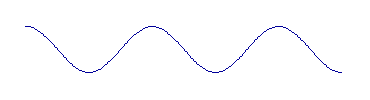# Wave-Particle Duality: Electrons

And so something that physicists had long considered to be simply a wave, light, turned out to behave like particles. The next question, asked by physicist Louis de Broglie, was "If waves can behave like particles, can particles behave like waves?" In the case of light, exposing the particle properties was simply a matter of creating the right circumstances (such as the photoelectric effect). The right circumstances for observing wavelike properties of electrons was created by physicists Davisson and Germer.

These guys aimed a beam of electrons at a crystal, and observed the electrons that were reflected off it. Now a crystal consists of atoms arranged in nice straight rows (or some other very orderly pattern). If the electron beam can behave as a wave, then as the wave is reflected off the crystal, the rows of atoms should have the same effect as the slits in Young's experiment. The result is that instead of the electrons being scattered from the crystal randomly, the reflected electrons exhibit an interference pattern like the light in Young's experiment.

Anyway, Davisson and Germer did the experiment, and this is exactly what they found. The electron beam was reflected like a wave, rather than like particles. In other words, they found, as de Broglie had speculated, that waveŁparticle duality is a property not only of light (photons), but of matter as well. Electrons, protons, alpha particles, and anything else that physicists might discover.

Now de Broglie's theory was more than just qualitative. De Broglie derived the mathematical relationships between the particle properties of a waveŁparticle, and its wave properties. When a physicist thinks of a wave, this is what he or she sees:The distance between the top of one wave and the top of the next (about two inches in the graphic above) is called the wavelength of the wave. If you pick a point on the graph above and count the waves as they go by, the number of waves per second is called the frequency of the wave. Planck derived a relationship between the frequency of a wave and the kinetic energy of the corresponding particle. (Like momentum, kinetic energy is a measure of how "hard" a particle is moving.) And de Broglie derived a relation between the momentum of a particle and the wavelength of the corresponding wave.

The greater the momentum, the shorter the wavelength.
The greater the kinetic energy, the higher the frequency.
This holds true for any atomic or subatomic particle, and in principle it holds for larger objects too, except that the wave properties of macroscopic objects are pretty much impossible to detect.
The meaning of the wave
Previous page
The Page of Uncertainty